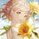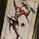701浏览
701
Introduction

The correlation oscillator is a technical indicator that measure the linear relationship between the market closing price and a simple increasing line, the indicator is in a (-1,1) range and rise when price is up-trending and fall when price is down-trending. Another characteristic of the indicator is its inherent smoothing which provide a noise free (to some extent) oscillator.

Such indicator use simple moving averages as well as estimates of the standard deviation for its calculation, but we can easily make it adaptive, this is why i propose this new technical indicator that create an adaptive correlation oscillator based on the Kaufman adaptive moving average .

The Indicator

The length parameter control the period window of the moving average, larger periods return smoother results while having a low kurtosis , which mean that values will remain around 1 or -1 a longer period of time. Pre-filtering apply a Kaufman adaptive moving average to the input, which allow for a smoother output.

No pre-filtering in orange, pre-filtering in yellow, period = 100 for both oscillators.

If you are not aware of the Kaufman adaptive moving average , such moving average return more reactive results when price is trending and smoother results when price is ranging, this also apply for the proposed indicator.

Conclusion

Classical correlation coefficients could use this approach, therefore the linear relationships between any variables could be measured. The fact that the indicator is adaptive add a certain potential, however such combination make the indicator have the drawback of kama + the correlation oscillator, which might appear at certain points.